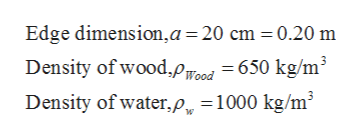# A cube of wood having an edge dimension of 20.0 cm and a density of 650. kg/m3 floats on water. (a) What is the distance from the horizontal top surface of the cube to the water level? (b) What mass of lead should be placed on the cube so that the top of the cube will be just level with the water surface?

Question
1 views

A cube of wood having an edge dimension of 20.0 cm and a density of 650. kg/m3 floats on water. (a) What is the distance from the horizontal top surface of the cube to the water level? (b) What mass of lead should be placed on the cube so that the top of the cube will be just level with the water surface?

check_circle

Step 1

Given,help_outlineImage TranscriptioncloseEdge dimension,a=20 cm = 0.20 m Density of wood,P rood = 650 kg/m³ Density of water,p„ =1000 kg/m fullscreen
Step 2

Part (a):

Cube of wood will submerge until weight of wood will be equal to submerged force. The sum of force after equilibrium is given as,

Step 3

By substituting the ...

### Want to see the full answer?

See Solution

#### Want to see this answer and more?

Solutions are written by subject experts who are available 24/7. Questions are typically answered within 1 hour.*

See Solution
*Response times may vary by subject and question.
Tagged in

### Fluid Mechanics